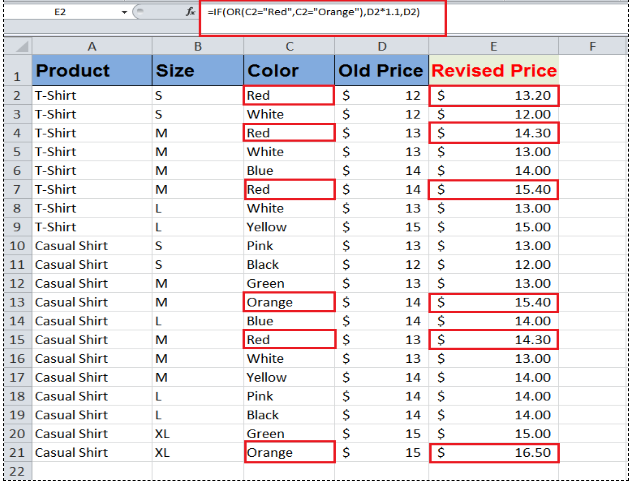Get instant live expert help with Excel or Google Sheets“My Excelchat expert helped me in less than 20 minutes, saving me what would have been 5 hours of work!”

#### Post your problem and you’ll get expert help in seconds.

Your message must be at least 40 characters
Our professional experts are available now. Your privacy is guaranteed.

# How to Use the IF and OR Functions in Excel

While evaluating a dataset, you might come across to a situation where you need to test multiple conditions or logical tests. Excel’s IF function allows users to logically compare values by testing condition(s) and returning a value if that condition(s) is TRUE or FALSE.

If you have multiple conditions to logically test and want to return a value where any one of the conditions is TRUE or FALSE then IF OR statement comes into action.

## Using Excel’s IF and OR functions

The Excel OR function is categorized as a logical function, and it returns TRUE if any of the given conditions are TRUE, otherwise, it returns FALSE.

As a combined IF OR statement, OR function is used as a logical_test argument and if any of the specified conditions are TRUE, OR function returns TRUE, and IF statement returns value_if_true argument’s value, otherwise it returns value_if_false argument’s value.

### The syntax of IF OR Statement;

`IF(OR( condition1, [condition2], ... ), value_if_true, value_if_false)`

Please note when you have entered all the conditions in the OR function then you must put a closing bracket to complete logical_test argument. After that, you need to enter TRUE or FALSE argument as part of the IF statement.

When any of the conditions are TRUE in an OR function, then you can perform calculations as per your requirement in TRUE or FALSE arguments of the IF OR formula in Excel, such as;

`=IF(OR(A2=”A”,B2<=20),C2*1.5,C2*1.2)`

In this IF OR formula, it says, if cell A2 value is “A” or cell B2 value is less than equal to 20, then return value after multiplying cell C2 value with 1.50, otherwise, return value after multiplying cell C2 value with 1.20. In this way, you can perform various calculations if any of the conditions are TRUE/FALSE.

### Example

Let’s suppose you have a dataset of men’s garments of a store. Each product has different prices for different sizes and colors available in stock. Management has decided to revise prices of products that come in any of the specified colors, irrespective of their sizes, and keep the rest of products’ prices same.

Using the IF OR statement, you can specify the colors whose prices are to be revised with a certain percentage say by 10% and keep the rest of the products’ prices the same as previous. Let say any product that comes in any of colors “Red” and “Orange” the prices of those products to be increased by 10% and keep the rest of the products’ prices the same.

You need to supply these two conditions in OR function of IF OR formula. OR function will return TRUE, if the product’s color meets any of these colors (Red and Orange), and IF statement returns revised price of that product after multiplying the old price with 1.10 as specified in value_if_true argument, otherwise IF statement will return old price of that product.

IF OR statement for the above example would be as follows;

`=IF(OR(C2="Red",C2="Orange"),D2*1.1,D2)`Here you can see that IF OR formula has performed the required calculations where any of the given conditions are TRUE otherwise it has returned the old values of products’ prices.

Still need some help with Excel formatting or have other questions about Excel? Connect with a live Excel expert here for some 1 on 1 help. Your first session is always free.

Solution examplesI have a list or people who are ranked in numbers from 3 to 6 I need to recognize the contents of each multiple cells and create an equivalent letter. for that value into another column. e.g. Cell E1 = 3 to show in new cell that row (H1) the letter "C" I have multiple rows with different values in column "E" Can this be done?
Solved by I. J. in 30 minsCould you help me by explaining the IF function?
Solved by G. L. in 24 minsI have a question about Conditional Formatting. =IF('Sheet1'!A1="X",IF('Sheet1'!B1="Y", TRUE, FALSE),FALSE) the set the cell background to red. I would like to make it so that if Sheet1'!B1="Z" I set the back to blue. How can I achieve this.
Solved by B. F. in 39 minsI need to find out if the 2018 yearly sales goals were met if the yearly sales were \$25,000 or more using an IF logical function and to set the formula to return a value of YES if met, and NO if not
Solved by A. A. in 18 minsHello good afternoon, I have a problem with excel. I need a formula to compare data between 3 columns and give me the result in a fourth column
Solved by X. B. in 58 mins